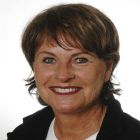Kategorien Preise UnternehmenKostenloses Lehrbuch

# Basic Thermodynamics: Software Solutions – Part I

14 Bewertungen
(14 Bewertungen)
1 Review
218
Sprache:  English
This book contains Solutions to Problems in Basic Thermodynamics, as per the syllabus of B.E. courses in Visweswaraya Technological University (VTU), Karnataka, India (and other Universities as well).
Beschreibung
Inhalt
Bewertungen

This book contains Solutions to Problems in Basic Thermodynamics, as per the syllabus of B.E. courses in Visweswaraya Technological University (VTU), Karnataka, India (and other Universities as well).

In this book, problems are solved using three popular software, viz. “Mathcad”, “Engineering Equation Solver (EES)” and “The Expert System on Thermodynamics (TEST)”. Some ‘free software’ are also explained.

An introductory chapter gives a brief overview of the software used.

The book is presented in four Parts, and this is Part-I:

Part-I contains an Introduction to software used, information and worked-out problems on the topics of Units, Pressure, Temperature, and examples of extensive use of software to find properties of Pure substances and to perform related calculations.

1. Introduction To The Software Used
1. Introduction
3. ‘Free’ Software
4. Summary
5. References
2. SI units, Unit conversion, Pressure, Temperature etc
1. Introduction
2. International System of Units (SI)
3. Conversion of Units
4. Examples of Unit conversion
5. Examples of Pressure calculations with Manometers
6. Examples of Temperature calculations with Thermocouples
7. Constant volume gas thermometer
8. Resistance Thermometer Detectors (RTD)
9. Summary
10. References
3. Properties Of Pure Substances
1. Introduction
2. Property diagrams for Water
3. Property Diagrams from Software
4. Property values and Tables
5. Example Problems
6. Determination of ‘quality’ (or dryness fraction) of wet steam
7. Conclusion
8. References
9. Exercise Problems
This book is very good for second/third year Mechanical Engineering students. The solutions for the various problems are excellent. The Mathcad software is used very efficiently and intelligently for solving the problems.. I am using this book for my research work sponsored by ASHRAE.

Walter Jaburek

Janine du PlessisChristian JulmiErik BruunDavid Shindler

Yuri YevdokimovMatthias KohlChristopher J. Skousen